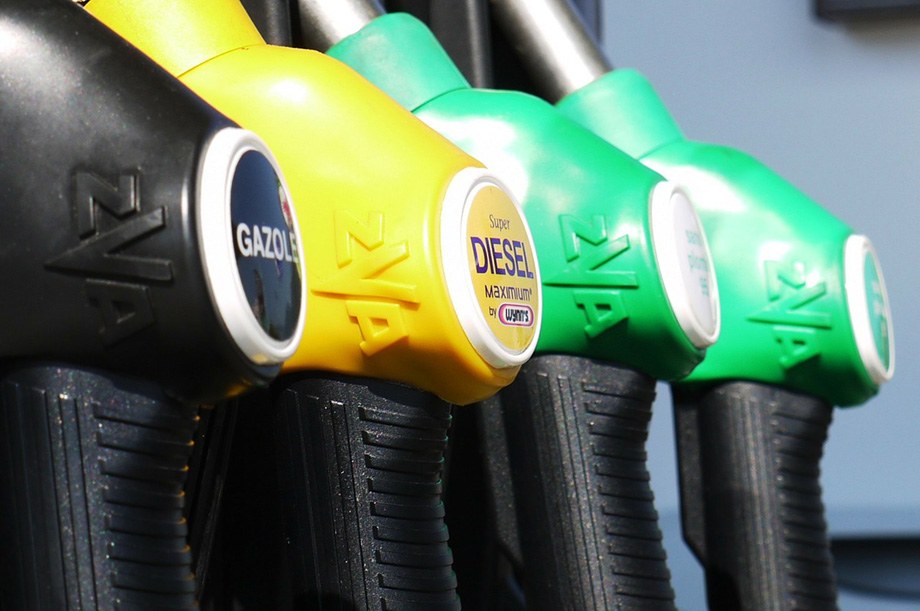# Fuel Consumption Converter

Use this fuel consumption converter to easily convert your fuel consumption rate from one unit to another. Metric and imperial units are supported, including miles per gallon, gallons per mile, km per litre, litres per 100 kilometers, gallons per 100 miles, etc.

Share converter:

Embed this tool:
get code

## Conversion of Fuel Consumption

Different countries have adopted different fuel consumption measurement units depending on the local units for measuring volume (liter/litre, U.S. gallon, U.K. gallon) and whether the measurement is present as the fuel needed to cover a specified distance, e.g. liters per 100 km or gallons per 100 miles, or whether it is presented as miles one can cover on a single liter or gallon (miles per gallon, kilometers per liter, etc.). It should be noted that the relation between units of the same type is linear, but it is not so if one converts from one type to another.

Our free online fuel consumption converter supports 12 different units: MPG (miles per gallon, US or UK), miles per liter (mi/L), km per liter (km/L), liters per 100 km (L/100km), liters per 100 miles (L/100mi), km per gallon (US or UK), gallons per 100 miles (US or UK), and gallons per 100 kilometers (Gallons/100km US or UK). The tool supports conversion between any pair of these units. The definitions of the units follow the international measurement conventions .## Measuring Fuel Consumption

Measuring fuel consumption and calculating the fuel efficiency (a.k.a. fuel economy) or a vehicle can be a complex thing to accomplish, depending on the final goal. Say one wants to compare two types of cars: A and B. We take two researches, one American and one French, and task them with choosing the car with the best fuel efficiency.

The first researches decides to use miles per gallon while the second decides to go with gallons per 100 miles. Each researcher records the efficiency of 2 cars of each type or the consumption of one and the same car in two different environments (the data available is the same for both), and the results look like so:

Fuel consumption of car type A:

Researcher Car 1 Car 2 Average
American (miles per gallon) 1 mpg 4 mpg 2.5 mpg/l
French (gallons per 100 miles) 100 g/100mi 25 g/100mi 62.5 g/100mi

Fuel consumption of car type B:

Researcher Car 1 Car 2 Average
American (miles per gallon) 2 mpg 2 mpg 2 mpg
French (gallons per 100 miles) 50 g/100mi 50 g/100mi 50 g/100mi

The numbers are obviously artificial to keep the math as simple as possible. What is important is that each has the same data, it is only expressed in different fuel consumption units, the numbers are equivalent when converted one way or the other. The US engineer will conclude from the above tables that cars of type A are more efficient than type B as they can cover a greater distance with a gallon of fuel (for type A the average is 2.5 while for type B it is just 2.0). The other engineer will conclude that cars of type B are more efficient as the car will use less fuel to cover the same distance (for type A the mean is 62.5 while for type B it is 50). Amusingly, the two researchers will arrive at different conclusions simply because they are using different measurement units! You can easily check that the values in the tables are otherwise exact conversions, e.g. 1 mpg equals exactly 100 gallons per 100 miles.

How do we decide which approach is correct? If we care about how much fuel we would need to cover a given distance the French researcher would be correct since the average is misleading the US researcher. Here is why: if you were to take both cars of type A and want to cover 100 kilometers with each of them, you would need not 200 / 2.5 = 80 gallons, but 125 gallons of petrol (100 gallons for the first car and 25 gallons for the second). The French will get it right by multiplying 200 x 0.625 = 125. For type B both researchers will correctly estimate that 100 gallons will be required for the same task. Therefore, type B is more efficient than type A.

However, there is another way to define fuel efficiency: as covering the furthest distance given a certain fuel amount. Under this definition the US researcher would be correct since if we give each car of type A a gallon of fuel, they will cover 5 miles combined while giving each car of type B a gallon of fuel, they will cover only 4 miles combined.

The above is an illustration of why when using our fuel consumption converter to make such calls we must always keep track of the end goal and define the question precisely. It is also a lesson on applying appropriate data transformations: the arithmetic average is not invariant to monotonic transformations so any conversions should be undertaken with utmost care. The above example uses as basis one from Hand D. (1994) "Deconstructing Statistical Questions", Journal of the Royal Statistical Society, Series A, Vol.157-3:317-356.